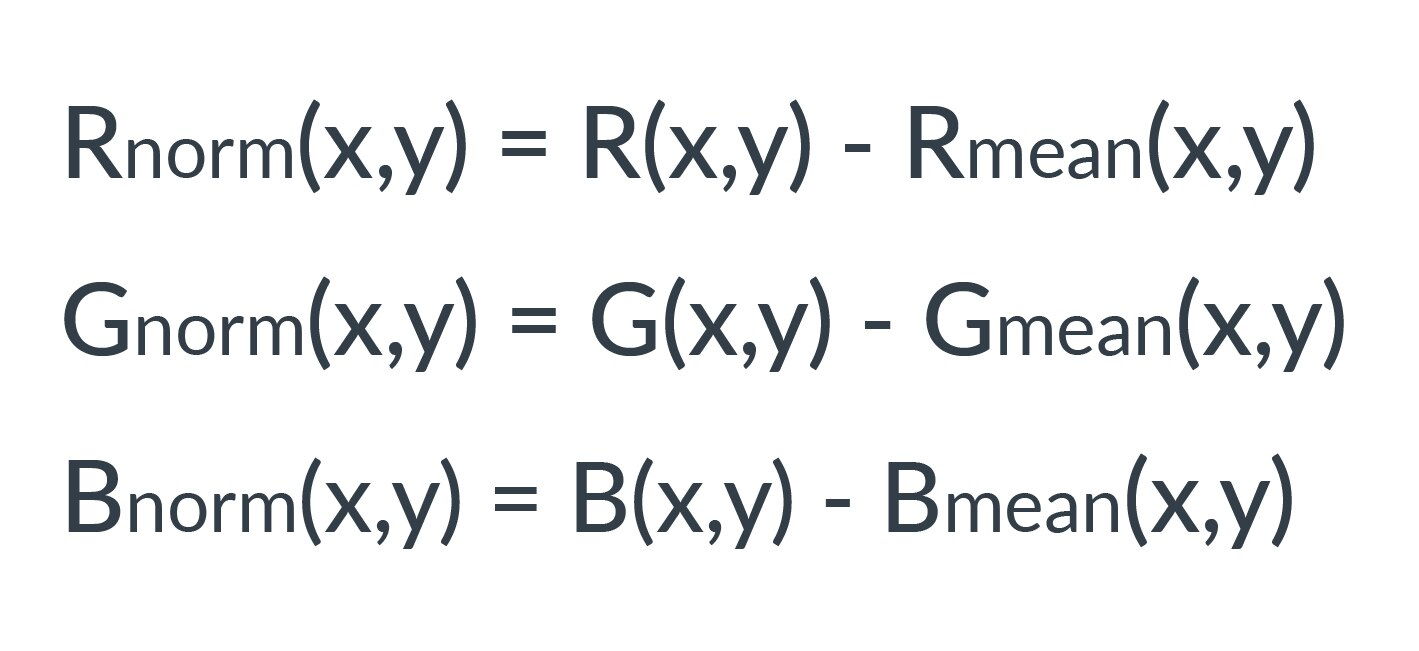## Evaluate the example code

A C++ implementation of AlexNet using the Graph API is proposed in examples/graph_alexnet.cpp. Here, we look at some key elements of the code and what they do.

To run the AlexNet example, you need to include these four command-line arguments:

```./graph_alexnet <target> <path_cnn_data> <input_image> <labels>
```

Where:

1. Target is the type of acceleration (`NEON=0` or `OpenCL=1`).
2. Path to cnn_data.
3. Path to your input image. Note that only .ppm files are supported).
4. Path to your ImageNet labels.

These subsections describe the key aspects of the example:

#### Mean subtraction pre-processing

A pre-processing stage is needed for preparing the input RGB image before feeding the network, so we are going to subtract the channel means from each individual color channel. This operation centers the red, green, and blue channels around the origin:Where:

• r_norm(x,y), g_norm(x,y), b_norm(x,y) are the RGB values at coordinates x,y after the mean subtraction.
• r(x,y), g(x,y), b(x,y) are the RGB values at coordinates x,y before the mean subtraction.
• r_mean, g_mean and b_mean are the mean values to use for the RGB channels.

For simplicity, the mean values for the examples are already hard-coded as:

`const std::array<float, 3=""> mean_rgb{ { 122.68f, 116.67f, 104.01f } }; </float,>`

If you are not familiar with mean subtraction pre-processing before, the Compute Image Mean section on the Caffe website provides a useful explanation.

#### Network description

The body of the network is described through the Graph API.

The graph consists of three main parts:

1. Mandatory: One input "Tensor object". This layer describes the geometry of the input data along with the data type to use. In this case, we will have a 3D input image with shape 227x227x3, using the FP32 data type.
2. The Convolution Neural Network layers, or ‘nodes’ in the graph's terminology. These are needed for the network.
3. Mandatory: One output "Tensor object". This is used to get the result back from the network.

As you can see in the example, the Tensor objects (input and output) and all of the trainable layers accept an input function called accessor.

Important: The accessor is the only way to access the internal Tensors.

• The accessor used by the input Tensor object can initialize the input Tensor of the network. This function can also be responsible for the mean subtraction pre-processing and reading the input image from a file or camera.
• The accessor used by the trainable layers, such as convolution, fully connected, and so on, can initialize the weights and the biases reading. For example, the values from a NumPy file.
• The accessor used by the output Tensor object can return the result of the classification, along with the score.

If you want to understand how the accessor works, see the utils/GraphUtils.h file. This has some ready-to-use accessors for your Tensor objects and trainable layers.